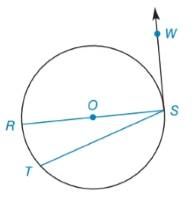Chapter 6.2, Problem 5EElementary Geometry For College St...

7th Edition
Alexander + 2 others
ISBN: 9781337614085

Solutions

Chapter
SectionElementary Geometry For College St...

7th Edition
Alexander + 2 others
ISBN: 9781337614085
Textbook Problem

Find m ∠ R S T if m R T ⌢ : m T S ⌢ = 1 : 4To determine

To find:

mRST if mRT:mTS=1:4.

Explanation

Definition:

An angle in a semi-circle is supplementary.

Corollary:

The measure of an angle formed by a tangent and a chord drawn to the point of tangency is one-half the measure of the intercepted arc.

Calculation:

To find: mRT.

Diameter is RS¯ and tangent is SW.

mRT:mTS=1:4

mRTmTS=14mTS=4mRT

By definition,

mRT+m<

Still sussing out bartleby?

Check out a sample textbook solution.

See a sample solution

The Solution to Your Study Problems

Bartleby provides explanations to thousands of textbook problems written by our experts, many with advanced degrees!

Get Started

In Exercises 11-16, find the slope of the line that passes through each pair of points. 12. (4, 5) and (3, 8)

Applied Calculus for the Managerial, Life, and Social Sciences: A Brief Approach

30. The random variable x has a normal distribution with and . Find B so that

Mathematical Applications for the Management, Life, and Social Sciences

0 1 ∞

Study Guide for Stewart's Multivariable Calculus, 8th

Rectangular coordinates of the point with polar coordinates are: (−1, 0) (0, 1) (0, −1) (1, 0)

Study Guide for Stewart's Single Variable Calculus: Early Transcendentals, 8th